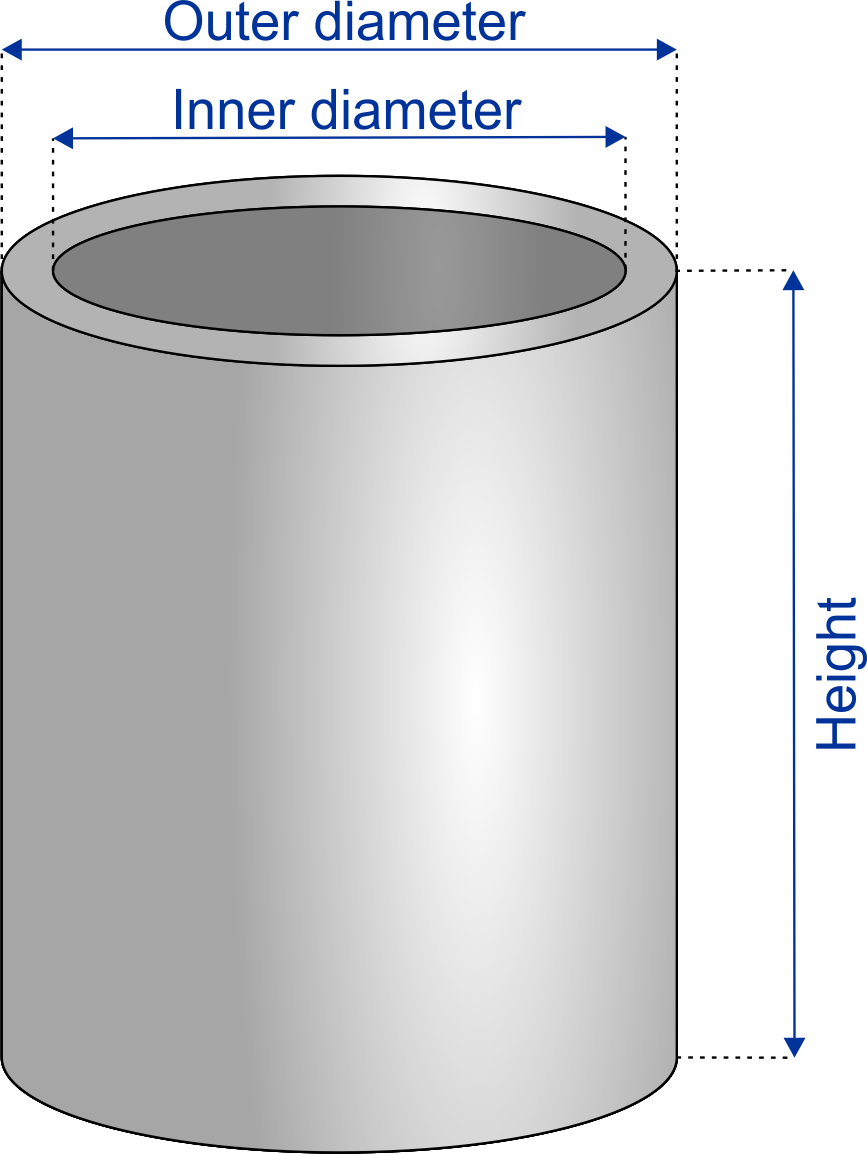# Concrete Estimator - Tube

Created by Mateusz Mucha and Joanna Andrzejewska
Reviewed by Jack Bowater
Last updated: Feb 27, 2022

This concrete tube estimator lets you estimate how many bags of cement you will need to make a tube shaped concrete element. If the cement you intend to buy is sold in bags, and if you know the size of the bag, our calculator will help you decide how many bags you need to buy for your project. All you need is a couple of dimensions. Our concrete calculator will also figure out the overall volume and weight of your concrete tubes. If you would like to estimate the amount of cement you need for different shaped elements, check our sibling concrete calculators for columns and slabs.

## How to figure out concrete

To figure out how much concrete you need to buy you simply need to multiply your tubes volume by its density which will determine its weight. It is a little more complicated for tubes but you can calculate it easily using our concrete estimator. This is how it's done:

1. First, you need to decide on the dimensions of your tube shaped element. Provide the outer and inner diameters, and the hight of the tube.
2. Determine the number of tubes you need.
3. Based on this data our concrete - tube estimator will count the overall volume of all those elements.
4. Provide the density of cement you will use (cement is usually sold in bags, therefore you will find this information on the bag). We put in a common value, but it can vary. You can also check out how to calculate density here.
5. Specify the weight of one bag of cement - again check on the bag.
6. Account for waste and spillage (5-10% usually) and there you have it - the number of bags you really need.

All dimensions are given in default units. You can change them to any other unit by simply unlocking the field (the padlock button).

## An example calculation

So let's say we have 5 concrete tubes with an inner diameter of 1.3 ft, an outer diameter of 1.6 ft, and a height of 8 feet. Construction elements are usually measured in feet but pre-mixed concrete is usually sold by the cubic yard, therefore our default volume unit is cubic yards. In our example, all of our tubes come to 1,01 cu yd (27,33 cubic feet).

Once we have the above measurements all that is left to do is to:

• Check if the density of cement from a particular producer is the same as the default value in our calculator (150 lb/cu ft). Feel free to change it as you need.
• Now you know the total weight of the required concrete,
• Check the weight of a single bag of cement - our default is 60 lb. Again, if not this is not the case, change it to appropriate weight.
• To be safe, account for spillage and waste. Experienced contractors usually assume 5-10%.

In our example we'll calculate using the default measures. We need 1,01 cubic yards of cement, our producer sells cement in bags weighing 60 lb with a 150 lb/cu ft density. The total weight of concrete that we need is 4100,04 lbs. We assume a 5% loss of waste and spillage, now we know that we need 72 bags of cement.

## How much is a yard of concrete?

A yard of concrete is simply a cubic yard defined as the volume of a cube with sides of 1 yard. A yard is equal to 3 feet or 36 inches. Mixed concrete is sold by cubic yards - therefore this is our default unit of volume.

Mateusz Mucha and Joanna Andrzejewska
ElementsOuter diameter
ft
Inner diameter
ft
Height
ft
Quantity
pieces
Volume
cu yd
Pre-mixed concrete
Density
lb/cu ft
Weight
lb
Bag size
lb
Waste
%
Bags needed
Costs
$/bag$/tube
$/ cu yd Total cost$
If you want to estimate how much reinforcement you need, check out our rebar calculator.
People also viewed…

### Body fat

Use the body fat calculator to estimate what percentage of your body weight comprises of body fat.

### Car vs. Bike

Everyone knows that biking is awesome, but only this Car vs. Bike Calculator turns biking hours into trees! 🌳

### DIY shed cost

Use our DIY shed cost calculator to estimate the cost of building a DIY shed. It'll help you decide on its size, roof type, and overhang length, and find the span of the roof rafter required.

### Gallons per square foot

Use this gallon per square foot calculator to determine how many gallons of liquid fit one square foot over a given depth.